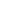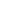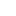# Moore Research Center, Inc.

•••When tax codes were revised in the early 1980's to change regulations governing capital gains/losses, volume and open interest in deferred futures contracts were considerably deflated as interest in spreads declined sharply. For example, traders had been doing such things as buying May Silver and selling March Silver as a spread in June, taking a loss on the the losing side on December 31 in order to claim a loss for their taxes for that year, then taking the gain on January 2 to claim a capital gain for the next year.

In addition, few commodity funds and pools trade spreads because trading systems are designed most typically for outright positions. Newer traders have little background or source of reference for spreads. Thus, except for commercials and some professional traders, spreads have been relegated more to the backwaters of the industry. However much ignored, they remain both a lower-risk trading vehicle and a great source of analytical information -- all the while retaining a certain elegance amidst the cacophony of the marketplace.

## Some Basics

Spreads are harder to track. Some of their concepts can be hard to grasp initially.

For instance, a spread being long March and short May Wheat may seem viable. If the May contract is at a higher price than the March, the spread is trading at a negative number (subtracting the price of the sell side from the price of the buy side). However, does one want the spread, then, to widen or narrow? (Because a spreader wants the long side to increase in value relative to the short side, he wants spread values to constantly become more positive. In this case, he wants the spread first to narrow toward 0 and then widen to more positive values.)

How does one quote a spread? One of two ways is easiest. Assume March Wheat is priced at \$4.50 and May Wheat at \$4.60. One may quote the March/May Wheat spread as trading at minus 10 cents (always subtracting the sell side from the buy side); or one may refer to it as trading at 10 cents premium to the May, or even as 10 cents premium to the sell side.

Although most spreads are quoted as buying one contract and selling another, there are some exceptions. In the financial area, certain spreads between various interest rate instruments are so standard as to be referred to in shorthand, as if the spread were a single entity. For instance, one buys the TED spread (T-Bills/ Euro Dollars) or one sells the TED spread but does not buy Euros and sell T-Bills. The same is true of the MOB and the NOB.

Another problem is computing the relationship of two futures contracts which have differing values per price tick. If the relationship is calculated as merely the difference of the prices, then the answer can be misleading. At minimum, it requires more calculation before a trader can assess profit or loss.

This problem can be illustrated with the following example. Assume a Heating Oil/Crude Oil spread, entered when the price of Heating Oil is 5600 and the price of Crude Oil is 2100. If the price of each contract rises 100 ticks, although the price difference between them remains the same, the spread actually loses\$580 in equity. However, if the price of each declines 100 ticks, again maintaining the same difference in price, the spread actually gains \$580 in equity. In each case the original price differential remained unchanged, but the relative equity of the contracts changed dramatically.

This situation does not exist if the dollar per tick value for the two contracts is the same. For example, assume a T-bond/T-note spread is entered with the price of bonds at 98-00 and the price of notes at 95-00. If the price of each goes up 16 ticks, the relative equity of the spread remains even (16 x \$31.25 = 16 x \$31.25).

To alleviate the problem in spreads wherein tick values are not equal, one can convert the price of each contract into an equity value for each contract. Therefore, in the Heating Oil/Crude Oil example (in which a dollar move in Heating Oil is worth \$420 and one in Crude Oil is \$1000), the entry price of the spread may be computed as follows:

 56.00 x 420 = 23,520 21.00 x 1000 = 21,000 equals 2,520

If the price of both were to rise 100 ticks, the equity value of the spread would be:

Thus, the equity of the spread declined from \$2520 to \$1940 with a resultant loss of \$580.

 57.00 x 420 = 23,940 22.00 x 1000 = 22,000 equals 1,940

Conversely, if the price of both declined 100 ticks, the equity becomes:

In this instance, the spread rose in value by \$580, from \$2520 to \$3100.

 55.00 x 420 = 23,100 20.00 x 1000 = 20,000 equals 3,100

In both cases a chart of price differences only would display a constant value, inaccurately implying no loss or gain in trade equity. Therefore, to track the progress of an equity spread one must constantly compute the relative equity value. After all, knowing whether a trade is making or losing money is the cornerstone of good money management.

Last Updated on Thursday, 22 May 2014 05:09